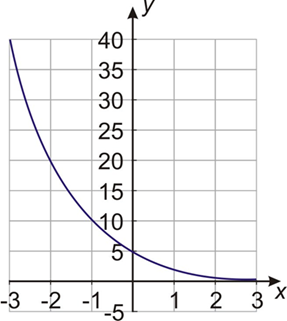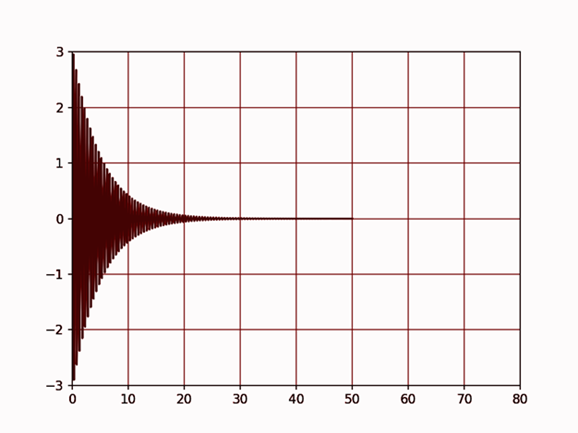# How to plot Exponentially Decaying Function using FuncAnimation in Matplotlib

Machine LearningServer Side ProgrammingProgrammingMatplotlib

Let us assume that we want to animate a nature of function which is exponentially decaying like y = a(b)^x where b = growth factor and a = initial value.

An exponentially decay function would look like this,However, for now, we want to animate and plot the exponentially decaying tan function.

First import the libraries,

import numpy as np
import matplotlib.pyplot as plt
from matplotlib.animation import FuncAnimation

Define the axes,

fig, a = plt.subplots()

Plotting a blank figure with axes,

xdata, ydata = [], []
line, = ax.plot(xdata, ydata)

Set the limit of the grids,

ax.set_xlim(0, 10)
ax.set_ylim(-3.0, 3.0)
ax.grid()   

Define the function to generate the data which will be used in place of number of frames,

def frame_generator(i=0):
while i < 50:
i += 0.1
yield x, np.tan(2*np.pi*x) * np.exp(-x/5.)

Define function to plot the animation,

def animate(data):
x, y = data
xdata.append(x)
ydata.append(y)
xmin, xmax = ax.get_xlim()
if x >= xmax:
ax.set_xlim(xmin, 2*xmax)
ax.figure.canvas.draw()
line.set_data(xdata, ydata)
return line

Activate the animation,

ani = FuncAnimation(fig, animate, frame_gen, blit=True, interval=2, repeat=False)

Plot the graph,

plt.show()

## Output